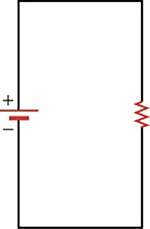Physics

# Extended Response

PhysicsExtended Response

### Extended Response

#### 19.1Ohm's law

61.
Explain how current and charge are related, including how direction is defined.
1. Current is the ratio of charge passing through a conductor per unit time. The current’s direction is the direction in which protons would flow.
2. Charge is the ratio of current passing through a conductor per unit time. The charge’s direction is the direction in which protons would flow.
3. Current is the product of charge passing through a conductor and the amount of time that passes. The current’s direction is the direction in which electrons would flow.
4. Charge is the product of current passing through a conductor and time. The charge’s direction is the direction in which electrons would flow.
62.
Under what conditions might a resistor material that normally follows Ohm’s law become non-ohmic?
1. If the amount of current flowing through the material is too low, the resistor may heat up, creating a nonlinear relationship between current and voltage.
2. If the amount of current flowing though the material is too high, the resistor may heat up, creating a nonlinear relationship between current and voltage.
3. If the amount of current flowing through the material is too low, the resistor may not warm up enough to allow a nonlinear relationship between current and voltage.
4. If the amount of current flowing through the material is too high, the resistor may not warm up enough to allow a nonlinear relationship between current and voltage.
63.
You connect a single resistor R across a 10\text{-V} battery and find that 0.01\,\text{A} flows through the circuit. You add another resistor R after the first resistor and find that 0.005\,\text{A} flows through the circuit. If you have 10 resistors R connected in a line one after the other, what would be their total resistance?
1. \frac{R}{10}
2. 5\,\text{R}
3. \frac{10}{R}
4. 10\,\text{R}

#### 19.2Series Circuits

64.

Explain why the current is the same at all points in the circuit below.1. If the current were not constant, the mobile charges would bunch up in places, which means that the voltage would decrease at that point. A lower voltage at some point would push the current in the direction that further decreases the voltage.
2. If the current were not constant, the mobile charges would bunch up in places, which means that the voltage would increase at that point. But a higher voltage at some point would push the current in the direction that decreases the voltage.
3. If the current were not constant, the mobile charges would bunch up in places, which mean that the voltage would increase at that point. A higher voltage at some point would push the current in the direction that further increases the voltage.
4. If the current were not constant, the mobile charges would bunch up in places, which mean that the voltage would decrease at that point. But a lower voltage at some point would push the current in the direction that increases the voltage.
65.

What is the current through each resistor in the circuit?1. Current through resistors R1, R2, R3, and R4 is 0.48 A, 0.30 A, 1.2 A, and 0.24 A, respectively.
2. Current through resistors R1, R2, R3, and R4 is 1200 A, 1920 A, 480 A, and 2400 A, respectively.
3. Current through resistors R1, R2, R3, and is R4 2.08 A, 3.34 A, 0.833 A, and 4.17 A, respectively.
4. The same amount of current, 0.096 A, flows through all of the resistors.

#### 19.3Parallel Circuits

66.
Power is supplied to a circuit in a house by one incoming wire at high potential compared with the ground. How does the voltage at each outlet on the circuit change and why?
1. All outlets on the circuit have the same voltage because they are wired in parallel.
2. All outlets on the circuit have the same voltage because they are wired in series.
3. Outlets further away from the source have a lower voltage because they are wired in parallel.
4. Outlets further away from the source have a lower voltage because they are wired in series.

#### 19.4Electric Power

67.
A single resistor is connected across the terminals of a battery When you attach a second resistor in parallel with the first, does the power dissipated by the system change?
1. No, the power dissipated remain same.
2. Yes, the power dissipated increases.
3. Yes, the power dissipated decreases.
68.
In a flashlight, the batteries are normally connected in series. Why are they not connected in parallel?
1. Batteries are connected in series for higher voltage and power output.
2. Batteries are connected in series for lower voltage and power output.
3. Batteries are connected in series so that power output is a much lower for the same amount of voltage.
4. Batteries are connected in series to reduce the overall loss of energy from the circuit.
Order a print copy

As an Amazon Associate we earn from qualifying purchases.